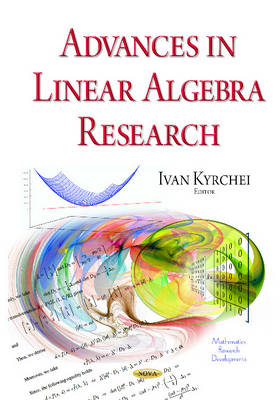•# Advances in Linear Algebra Research (Hardback)

(editor)
£221.99
Hardback 333 Pages / Published: 01/01/2015
• We can order this from the publisher

Usually dispatched within 15 working days

• This item has been added to your basket
This book presents original studies on the leading edge of linear algebra. Each chapter has been carefully selected in an attempt to present substantial research results across a broad spectrum. The main goal of Chapter One is to define and investigate the restricted generalized inverses corresponding to minimization of constrained quadratic form. As stated in Chapter Two, in systems and control theory, Linear Time Invariant (LTI) descriptor (Differential-Algebraic) systems are intimately related to the matrix pencil theory. A review of the most interesting properties of the Projective Equivalence and the Extended Hermite Equivalence classes is presented in the chapter. New determinantal representations of generalized inverse matrices based on their limit representations are introduced in Chapter Three. Using the obtained analogues of the adjoint matrix, Cramer's rules for the least squares solution with the minimum norm and for the Drazin inverse solution of singular linear systems have been obtained in the chapter. In Chapter Four, a very interesting application of linear algebra of commutative rings to systems theory, is explored. Chapter Five gives a comprehensive investigation to behaviors of a general Hermitian quadratic matrix-valued function by using ranks and inertias of matrices. In Chapter Six, the theory of triangular matrices (tables) is introduced. The main "characters" of the chapter are special triangular tables (which will be called triangular matrices) and their functions paradeterminants and parapermanents. The aim of Chapter Seven is to present the latest developments in iterative methods for solving linear matrix equations. The problems of existence of common eigenvectors and simultaneous triangularization of a pair of matrices over a principal ideal domain with quadratic minimal polynomials are investigated in Chapter Eight. Two approaches to define a noncommutative determinant (a determinant of a matrix with noncommutative elements) are considered in Chapter Nine. The last, Chapter 10, is an example of how the methods of linear algebra are used in natural sciences, particularly in chemistry. In this chapter, it is shown that in a First Order Chemical Kinetics Mechanisms matrix, all columns add to zero, all the diagonal elements are non-positive and all the other matrix entries are non-negative. As a result of this particular structure, the Gershgorin Circles Theorem can be applied to show that all the eigenvalues are negative or zero.

Publisher: Nova Science Publishers Inc
ISBN: 9781634635653
Number of pages: 333
Weight: 800 g
Dimensions: 260 x 180 mm

## Reviews

Sign In To Write A Review

Please sign in to write a review

Your review has been submitted successfully.